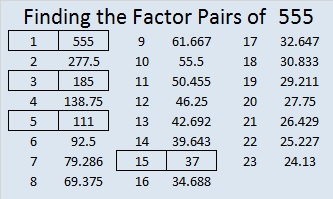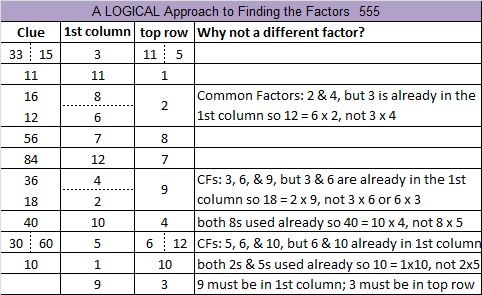# 555 and Level 5

555  is the hypotenuse of four Pythagorean triples:

• 171-528-555
• 180-525-555
• 312-459-555
• 333-444-555

Each of those Pythagorean triples has a greatest common factor. Can you figure out what each one is? Hint: each one will be a factor of 555 listed below the puzzle.Print the puzzles or type the solution on this excel file: 12 Factors 2015-07-13

—————————————————————————————————

• 555 is a composite number.
• Prime factorization: 555 = 3 x 5 x 37
• The exponents in the prime factorization are 1, 1, and 1. Adding one to each and multiplying we get (1 + 1)(1 + 1)(1 + 1) = 2 x 2 x 2 = 8. Therefore 555 has exactly 8 factors.
• Factors of 555: 1, 3, 5, 15, 37, 111, 185, 555
• Factor pairs: 555 = 1 x 555, 3 x 185, 5 x 111, or 15 x 37
• 555 has no square factors that allow its square root to be simplified. √555 ≈ 23.5584—————————————————————————————————